# Bulk acoustic resonator devices using ZnO-based film and back cavity

Bulk acoustic resonator devices using ZnO-based film and back cavity

Xin LiMengwei Liu Yanlu Feng

School of Information Science and Engineering, Shenyang University of Technology, Shenyang 110870, China

Institute of Acoustics, Chinese Academy of Science, Beijing 100190, China

Corresponding Author Email:
lixin97@163.com
Page:
111-125
|
DOI:
https://doi.org/10.3166/EJEE.19.111-125
|
Accepted:
|
Published:
30 April 2017
| Citation

OPEN ACCESS

Abstract:

The purpose of this study was to investigate bulk acoustic resonator (FBAR) devices using ZnO-based film and back cavity. A Mason equivalent circuit model was adopted to simulate the impedance characteristics of FBAR devices. The influence of piezoelectric material thickness, electrode thickness, and resonance area on the impedance characteristics of FBAR devices was analyzed. Structural parameters of the FBAR devices were designed, and bulk silicon micromachining was applied to fabricate Al/ZnO/Al-based FBAR devices with a back cavity. X-ray diffraction results revealed that ZnO piezoelectric films had a highly preferred c-axis orientation. The frequency response of longitudinal wave FBAR devices has been measured by an RF network analyzer, and the results indicated the series resonant frequency and parallel resonant frequency of the fabricated FBAR devices determined to be 1.546 GHz and 1.590 GHz, respectively, which were close to the simulated results. According to the measured results, the effective electromechanical coupling coefficient and the quality factor have been calculated to be 6.83% and 350, respectively. The findings of this study may serve as reference for the development of FBAR devices.

Keywords:

back cavity, bulk silicon micromachining, film bulk acoustic resonator, ZnO piezoelectric film

1. Introduction

The wireless communication technology has developed rapidly in recent years. Frequencies have increased from 500 MHz to 6 GHz, and the device circuitry has become progressively miniaturized and integrated. As important elements in RF circuits, filters have also had to shrink and integrate. Common surface acoustic wave filters and dielectric ceramic filters in common use no longer meet these requirements. The appearance of thin film acoustic resonator (FBAR) devices has led to their development (Lakin and Wang, 1981; Sang et al., 1999; Chen, 2010). Featuring high-frequency support, high Q, greater power capacity, and compatibility with CMOS-integrated circuit technology (Gao et al., 2016;), FBARs are important devices among next generation filters (Flewitt et al., 2015). FBAR devices have attracted the attention of manufacturers. However, design and manufacturing technology for the FBAR devices needs additional research.

This study is organized as follows. Section 2 presents working principle, device parameters and structure parameters of the FBAR. Section 3 discusses Mason equivalent circuit model, simulation, performance analysis and optimal design of FBAR device. Section 4 introduces the fabrication of FBAR device. Section 5 shows measurement results of an FBAR device. Section 6 summarizes the conclusions.

2. FBAR devices

2.1. Working principle

Body longitudinal acoustic waves and body shear acoustic waves can be excited in piezoelectric material. When acoustic waves propagate along the c-axis direction in the piezoelectric material, if the displacement direction of the acoustic wave particle is parallel to the wave propagation direction, the excited bulk acoustic waves are longitudinal. When the displacement direction of the acoustic wave particle is perpendicular to the wave propagation direction, the excited bulk acoustic waves are shear (Pang et al., 2012). Our FBAR device vibrates along the thickness direction with vibration belonging to the longitudinal wave mode (Mai and Lee, 2007). Thus, we neglect the influence of shear waves.

An ideal FBAR device is composed of a lower electrode, the piezoelectric film, and an upper electrode, as shown in Figure 1.

## 1.pngFigure 1. The structure diagram of an ideal FBAR

When an AC voltage signal V(f) is applied to the upper and lower electrodes of the device, the piezoelectric material between the two electrodes undergoes mechanical deformation due to the inverse piezoelectric effect. With a changing electric field, contraction and expansion of the piezoelectric film leads to vibration. The vibration produces bulk acoustic waves propagating along the thickness direction in the piezoelectric material. When the bulk acoustic waves propagate to the interface between either electrode and the air, they are reflected back. The waves thus bounce back and forth in the piezoelectric material forming oscillations. Thanks to the positive piezoelectric effect of the piezoelectric film, the oscillating acoustic waves produce a radio frequency signal. When the propagation distance of bulk acoustic waves in the piezoelectric film is equal to the half wavelength or an odd multiple of the half wavelength, the standing wave oscillation forms and produces resonance (Chen, 2005).

The electrical impedance characteristics of the FBAR are shown in Figure 2. The devices have a series resonant frequency fs and a parallel resonant frequency fp. When a given AC voltage is applied, the series resonant frequency fs of the device is obtained under the following conditions: the polarization vector P and the electrical field E are in phase within the piezoelectric film, the generated current is at maximum, and the electrical impedance of the FBAR is at minimum. The parallel resonant frequency fp is obtained when the polarization vector P and the electrical field E are completely out of phase, the generated current is at minimum, and the electrical impedance of the FBAR is at maximum.

## 2.pngFigure 2. Electrical impedance characteristics of an FBAR

2.2. The device parameters

In addition to fs and fp, the effective electromechanical coupling coefficient and the quality factor Q are two important parameters in evaluating the performance of the FBAR devices. These parameters are defined according to the equations (Liu, 2012).

$K _ { e f f } ^ { 2 } = \left( \frac { \pi } { 2 } \right) ^ { 2 } \frac { f _ { p } - f _ { s } } { f _ { p } }$      (1)

$Q _ { f _ { x } } = \frac { f _ { x } } { 2 } \left| \frac { \mathrm { d } \angle Z _ { i n } } { \mathrm { d } f } \right| _ { f _ { x } }$        (2)

The effective electromechanical coupling coefficient K2eff represents the conversion degree between the mechanical and electrical energy in the device. The bandwidth of an FBAR filter increases with increasing K2eff. K2eff depends primarily on the piezoelectric material with a secondary dependency on the thickness of the upper and the lower electrode layers.

The quality factor Q indicates the bulk acoustic wave loss in the FBAR and the insertion loss of the FBAR filter. The in-band insertion loss decreases with increasing Q. Q depends not only on the acoustic wave loss in the electrode and piezoelectric materials but also on the degree of confinement of the bulk acoustic waves within the sandwich structure. The loss of bulk acoustic waves in the material and the degree of leakage in the FBAR decreases with increasing Q. In actual production, the Q value is enhanced primarily by the high-quality manufacture and orientation of the piezoelectric film.

2.3. Structure of the FBAR

According to transmission line theory, the incident wave is reflected back when the load is infinite or zero. Thus, the acoustic impedance of the upper and lower boundary in the sandwich structure must be infinite or zero. Since the acoustic impedance of the air is approximately zero, it serves as the better acoustic limiting interface. Another acoustic limiting interface is a Bragg reflection layer composed of 1/4 wavelength deposition layers with alternating high and low acoustic impedances. The surface of the upper electrode in an FBAR device is usually in contact with the air. However, the lower surface of the FBAR device is usually in contact with a substrate material such as silicon making it necessary to form a better acoustic limited interface via the etching process.

MEMS micromachining etches away most of silicon on the back of the device, forming a back cavity and creating the interface between the air and the metal on the surface of the lower electrode. With air at both electrodes, the generated acoustic waves are confined in the sandwich structure. For silicon substrates, the etching process typically uses wet etching or Inductively Coupled Plasma. Either method removes most of the substrate when forming the FBAR device, yielding a device with poor mechanical strength. The devices are easily broken during production, decreasing the yield. To address this problem, low stress silicon nitride or silicon dioxide is deposited as a support layer below the lower electrode to improve mechanical rigidity at the expense of a reduced Q value (Park, 2003). Figure 3 shows an FBAR device with a back cavity.

## 3.png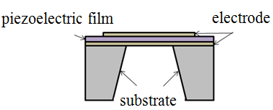Figure 3. An FBAR with back cavity

3. Device simulation and analysis

3.1. Mason equivalent circuit model

The general analytic expression for the impedance of the piezoelectric layer in an FBAR device is derived from acoustic theory and given in Formula 3 (Su et al., 2001).

$Z = \frac { 1 } { j \omega C _ { 0 } } \left[ 1 - k _ { t } ^ { 2 } \frac { \tan \theta } { \theta } \frac { \left( z _ { t } + z _ { b } \right) \cos ^ { 2 } \theta + j \sin 2 \theta } { \left( z _ { t } + z _ { b } \right) \cos 2 \theta + j \left( z _ { t } z _ { b } + 1 \right) \sin 2 \theta } \right]$       (3)

The above formula is properly mathematically processed. The transformed formula is expressed as

$Z=\frac{1}{j\omega {{C}_{0}}+\frac{1}{-\frac{1}{j\omega {{C}_{0}}}+{{n}^{2}}\left\{ -j{{Z}_{p}}\csc 2\theta +\frac{1}{\frac{1}{j{{Z}_{p}}\tan \theta +{{Z}_{t}}}+\frac{1}{j{{Z}_{p}}\tan \theta +{{Z}_{b}}}} \right\}}}$ (4)

where C0 is the static capacitance; θ is the phase displacement; k2t is the electromechanical coupling coefficient; and zt and zb are the normalized acoustic impedances at the upper and lower surfaces of the piezoelectric material, respectively. (Regarding the normalized acoustic impedances, zt=Zt/Zp and zb=Zb/Zp, where Zp is the characteristic acoustic impedance of the piezoelectric materials and Zt and Zb are the acoustic impedances at the upper and lower surfaces of the piezoelectric material, respectively.) Additionally, n2=2θ/k2twC0Zp represents the transformer in AC circuits. The usual symbols represent the inductance and the impedance. Therefore, Formula 4 can be represented with a Mason equivalent circuit for the piezoelectric material as shown in Figure4, with Zd=jZPtanq and Ze=jZPcsc2q. For an ideal FBAR device, Zt=Zb=0.

## 4.pngFigure 4. The Mason equivalent circuit of the piezoelectric layer

In the circuit, C0SzzA/2d and q =ωd/na, where A represents the effective area of FBAR, εSzz represents the clamped dielectric constant, 2d represents the thickness of the piezoelectric layer, and va represents the longitudinal wave velocity. From these values along with the characteristic acoustic impedance Zp and the acoustic impedances Zt and Zb, we obtain the Mason equivalent circuit of the corresponding piezoelectric layer.

In an FBAR device with a composite structure, the bulk acoustic waves propagate not only along the c-axis in the piezoelectric layer but also in the ordinary acoustic layer, the upper and lower electrode layers, the Bragg reflection layer, and the supporting layer. Therefore, electromagnetic transmission line theory can be adopted to express the transmission of acoustic waves in the ordinary acoustic layer. The transformer in Figure 4 represents the conversion between mechanical energy and electrical energy. Therefore, the Mason equivalent circuit of the ordinary acoustic layer assumes the form shown in Figure 5, where Zf and Zg represent the acoustic impedances, Zin represents the input impedance, and ZL represents the load acoustic impedance.

## 5.png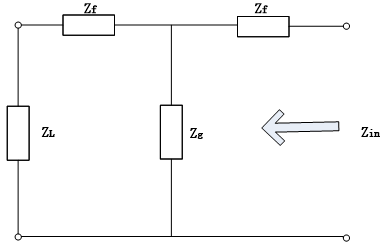Figure 5. The Mason equivalent circuit of the ordinary acoustic layer

The expression for Zin obtained from Figure 5 is

$Z _ { i n } = Z _ { f } + \frac { \left( Z _ { L } + Z _ { f } \right) Z _ { g } } { Z _ { L } + Z _ { f } + Z _ { g } }$ $= \frac { Z _ { L } \left( Z _ { f } + Z _ { g } \right) + Z _ { f } ^ { 2 } + 2 Z _ { f } Z _ { g } } { Z _ { L } + Z _ { f } + Z _ { g } }$   $= Z _ { 0 } \frac { Z _ { L } + \frac { Z _ { f } ^ { 2 } + 2 Z _ { f } Z _ { g } } { Z _ { f } + Z _ { g } } } { Z _ { 0 } + Z _ { L } \frac { Z _ { 0 } } { Z _ { f } + Z _ { g } } }$     (5)

and from transmission line theory, the expression for Zin is

$Z _ { i n } = Z _ { 0 } \frac { Z _ { L } + j Z _ { 0 } \tan ( k d ) } { Z _ { 0 } + j Z _ { L } \tan ( k d ) }$      (6)

where Z0 is the characteristic acoustic impedance, k is the acoustic transmission constant, and d is the thickness of the acoustic layer. Comparing Formulas (5) and formula (6), we obtain the formulas (7) and (8)

$\frac { Z _ { f } ^ { 2 } + 2 Z _ { f } Z _ { g } } { Z _ { f } + Z _ { g } } = j Z _ { 0 } \tan ( k d )$          (7)

$\frac { Z _ { 0 } } { Z _ { f } + Z _ { g } } = j \tan ( k d )$             (8)

Applying trigonometric transformations to Formulas (7) and (8), we obtain

$Z _ { f } = j Z _ { 0 } \tan \left( \frac { k d } { 2 } \right)$           (9)

$Z _ { g } = \frac { Z _ { 0 } } { j \sin ( k d ) }$              (10)

Figure 6 shows the Mason equivalent circuit diagram of an FBAR device where fs and fp are the series resonant frequency and the parallel resonant frequency of the FBAR devices, respectively; fx represents either the series resonant frequency or the parallel resonant frequency; and $\angle Z_{\text {in }}$ is the impedance phase in radians. The upper and lower electrode, piezoelectric, and supporting layers are cascaded together to obtain the Mason equivalent circuits. We can analyze different cascading forms depending on the requirements in actual simulation.

## 6.png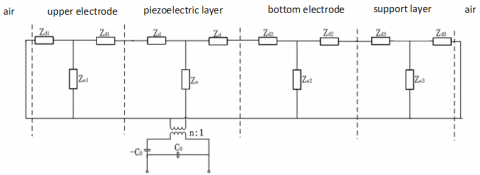Figure 6. The Mason equivalent circuit of the FBAR

3.2. FBAR simulation

We used the ADS (Advanced Design System) software from Agilent for our tests (Reinhardt et al., 2003). Figure 7 shows the ADS simulation scheme. The fabricated FBAR has an upper Al electrode layer, a ZnO piezoelectric layer, and an Al lower electrode layer. All layers are 0.1μm thick.

## 7.pngFigure 7. The ADS simulation of an actual FBAR

The simulation results of the impedance characteristics are shown in Figure 8. The thick line represents the amplitude frequency characteristic of the FBAR, and the fine line represents the phase frequency characteristic. The figure shows that the theoretical values of the series and parallel resonant frequencies of the FBAR device are 1.579GHz and 1.592GHz, respectively.

## 8.png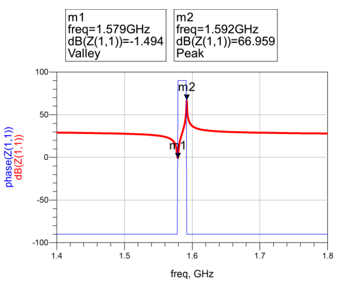Figure 8. Impedance characteristic of a fabricated FBAR

3.3. Analysis of FBAR performance

1) Influence of the piezoelectric material thickness on the impedance characteristics of an FBAR

The impedance characteristic curves of the FBAR with ZnO thicknesses of 0.7μm, 1μm, 1.3μm and 1.6μm are shown in Figure 9. The upper Al electrode layer thickness, lower Al electrode layer thickness and resonance area are 0.1μm, 1μm,200μm×200μm, respectively. The resonant frequency of the FBAR gradually decreases with the increase of the ZnO layer thickness.

## 9.pngFigure 9. Impedance of FBARs with ZnO of different thicknesses

2) Influence of the upper electrode thickness on the impedance characteristics of an FBAR

The impedance characteristic curves of an FBAR with upper Al electrode thicknesses of 0.1μm, 0.13μm, 0.16μm and 0.19μm are shown in Figure 10. The ZnO layer thickness, lower Al electrode layer thickness, and resonance area are 1μm, 1μm, and 200μm×200μm, respectively. Figure 10 shows that the resonant frequency of the FBAR decreases as the thickness of the upper Al electrode increases. The propagation path of the bulk acoustic waves increases with the increase of the electrode thickness, resulting in a decrease in the resonant frequency.

## 10.png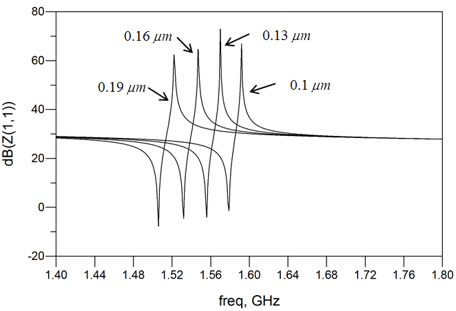Figure 10. Impedance of FBARs with electrodes of different thicknesses

3) Influence of the resonant area on the impedance characteristics of an FBAR

Figure 11 shows the impedance characteristic curves of FBAR devices with resonance areas of 200μm×200μm, 300μm×300μm and 400μm×400μm. The thicknesses of the upper Al electrode, ZnO piezoelectric layer, and lower Al electrode layers are all 0.1μm. From Figure11, one sees that the resonant frequency is independent of the resonance area. As the resonance area increases, the impedance of the non-resonance region decreases. The structure of the filter can be optimized using these FBAR performance characteristics.

## 11.pngFigure 11. Impedance of FBARs with different resonance areas

3.4. Optimal design of FBAR structure

According to the simulation analysis, the series and parallel resonance frequencies of the FBAR are related to the thickness of the piezoelectric material and electrodes. The ADS software determined the optimal thickness of the piezoelectric material, enabling calculation of the required series and parallel resonant frequencies of the FBAR.

The resonant frequency of our fabricated FBAR device is about 1.5GHz using a ZnO piezoelectric layer thickness of 2μm and upper and lower Al electrode thicknesses of 0.1μm and 1μm, respectively. We left the electrode thickness unchanged and optimized other parameters by changing the thickness of the ZnO piezoelectric layer. Figure 12 shows the impedance characteristic of an optimized FBAR device. The thickness of ZnO piezoelectric layer after optimization was 1.171μm with a series resonance frequency of 1.5 GHz. The thickness of the upper Al electrode layer, ZnO piezoelectric layer and lower Al electrode layer in the final design of the FBAR device are 0.1μm, 1μm and 1μm, respectively.

## 12.png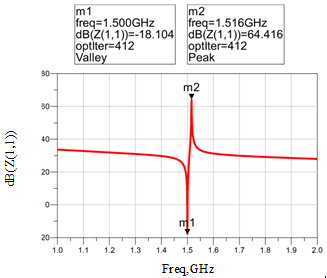Figure 12. The optimized impedance characteristic of an FBAR

4. Fabrication of FBAR devices

Figure 13 shows the manufacturing process for FBAR devices.

## 13.pngFigure 13. The manufacturing process of an FBAR

a) The silicon wafer is cleaned and dried.

b) An approximately 5000Å silicon nitride thin film is grown on the front side of silicon wafer by Plasma enhanced chemical vapor deposition, with the silicon nitride subsequently annealed to relieve the residual stress.

c) A layer of aluminum is grown on both sides of the silicon wafer using ion beam sputtering, with the positive aluminum layer forming the lower electrode, and the opposite layer used as the masking layer for dry etching.

d) Standard lithography is applied to etch the aluminum to form the lower electrode on the front side of the silicon wafer. The double side alignment process is used to define the etching window for Inductively Coupled Plasma on the back side of the silicon wafer, with concentrated phosphoric acid used to etch away the aluminum film in the window area.

e) The zinc oxide film is deposited on the lower electrode by magnetron sputtering, followed by photolithography.

f) The lift-off process is used to form the upper electrode on the zinc oxide piezoelectric material.

g) The front side of the wafer is protected while Inductively Coupled Plasma etching removes the needed large amount of silicon and silicon nitride from the back until the structure is completely released as the final FBAR device.

Aluminum and zinc oxide are used for the electrodes and piezoelectric layer, respectively. The use of Inductively Coupled Plasma etching for the back of the device solves the silicon fragility problem. The front and back of an FBAR with a circular electrode is shown in Figure 14. The small circle in the Figure 14a is the upper electrode of the FBAR, and the big circle is the lower electrode of the FBAR. The zinc oxide film is sandwiched between them.

## 14.pngFigure 14. A fabricated FBAR device

5. Results and analysis

The ZnO piezoelectric film in the FBAR devices vibrates along the thickness direction to form resonance, ZnO piezoelectric to be oriented along the c-axis. Figure 15 shows the X-ray Diffraction curve of ZnO film. When 2q=34.6, there is an obvious diffraction peak in the film indicating that ZnO piezoelectric films produced by sputtering have a high preference for orientation along the c-axis direction.

## 15.pngFigure 15. X-ray diffraction curves of ZnO film

The electrode of our FBAR was welded to the PCB board, and we used an Agilent E5071C RF network analyzer for measurement. The S22 test diagram of the amplitude frequency characteristic is shown in Figure 16. The resonance characteristics of our fabricated FBAR device are obvious.

## 16.png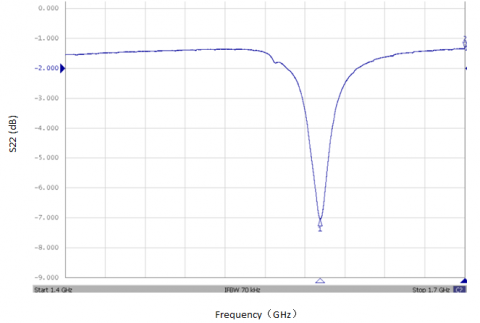Figure 16. The S22 measurement results of an FBAR device

The fabricated devices have two resonant frequencies: the series resonant frequency of 1.546 GHz and the parallel resonant frequency of 1.590 GHz, which are similar to the previous theoretical simulation results of 1.579 GHz and 1.592 GHz. According to Formulas (1) and (2) and the experimental data, K2eff and Q for our fabricated FBAR devices were calculated to be 6.83% and 350, respectively.

6. Conclusions

We analyzed the influence of the thickness of the ZnO thin film, the thickness of the electrodes, and the effective resonance area of the FBAR device on the impedance characteristics of the device. We also developed a suitable fabrication process for an FBAR device with a back cavity and used an Agilent E5071C RF network analyzer to measure a fabricated FBAR. The series and parallel resonant frequencies were both similar to the theoretical results obtained with software simulation. The good K2eff and Q values of the fabricated FBAR devices were expected. The RF filters and duplexers based on the FBAR devices will be used widely in wireless mobile communication systems.

Acknowledgments

This work is partially supported by the Liaoning Province Education Office (LFGD2017015). titre en italique, le reste en romain

References

Chen W. (2005). Research and modeling of thin film bulk acoustic wave filter FBAR. Ph.D. dissertation, Dept. Elect. Eng. Zhejiang University, Hangzhou. https://www.ixueshu.com/document/f49a4b3e4df97fa3318947a18e7f9386.html

Chen Y. C. (2010). Modeling of thin film bulk acoustic wave resonators and ladder-type filter design. Journal of Optoelectronics and Advanced Materials, Vol. 12, No. 9, pp. 1993-1999. https://doi.org/10.1142/9789812770165_0037

Flewitt A. J., Luo J. K., Fu Y. Q. (2015). ZnO based SAWand FBAR devices for bio-sensing applications. Non-Newton. Fluid Mech, No. 222, pp. 209-216. https://doi.org/10.1016/j.jnnfm.2014.12.002

Gao J., Liu G., Li J., Li G. (2016). Recent developments of film bulk acoustic resonators. Functional Materials Letters, Vol. 9, No. 3, pp. 163-173. https://doi.org/10.1142/S1793604716300024

Kim S. H., Lee J. S., Choi H. C., Lee Y. H. (1999). The fabrication of thin-film bulk acoustic wave resonators employing a ZnO/Si composite diaphragm structure using porous silicon layer etching. IEEE Electron Device Letters, Vol. 20, No. 3, pp. 113-115. https://doi.org/10.1109/55.748905.

Lakin K. M., Wang J. S. (1981). Acoustic bulk wave composite resonators. Appl. Phys.Lett, Vol. 38, No. 3, pp. 125–127. https://doi.org/10.1063/1.92298

Liu S. J. (2012). FBAR temperature sensor. Ph.D. dissertation, Dept. Elect. Eng. Zhejiang University, Hangzhou. http://cdmd.cnki.com.cn/Article/CDMD-10335-1012312433.htm

Mai L., Lee J. Y. (2007). Approach to improve ZnO-based FBAR devices. Electronics Letters, Vol. 43, No. 13, pp. 735-737. https://doi.org/10.1049/el:20070914

Pang W., Zhao H.Y., Kim E.S., Zhang H., Yu H.Y. (2012). Piezoelectric microelectromechanical resonant sensors for chemical and biological detection. Lab Chip, No. 12, pp. 29–44. https://doi.org/10.1039/c1lc20492k

Park J. Y., Lee H. C., Lee K. H. (2003). Micromachined FBAR RF filters for advanced handset applications. IEEE International Conference on Transducers Solid-state Sensors, Actuators & Microsystems, No. 1, pp. 911-914. https://doi.org/10.1109/SENSOR.2003.1215623

Reinhardt A., Laude V., Robert L. (2003). Numerical simulation of FBAR’s. WCU 2003, Paris, Vol. 1, pp. 1487-1494. https://doi.org/10.1109/ULTSYM.2002.1193450

Su Q. X., Kirby P., Komuro E., Imura M. (2001). Thin-film bulk acoustic resonators and filters using ZnO and Lead-Zirconium-Titanate thin film. IEEE Transactions on Microwave Theory and Techniques, Vol. 49, No. 4, pp. 769-778. https://doi.org/10.1109/22.915462